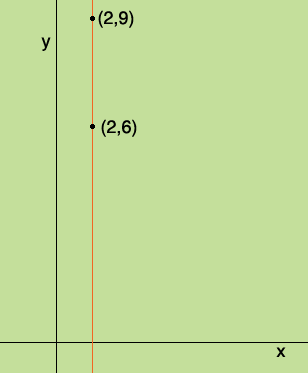SEARCH HOMEMath Central Quandaries & QueriesQuestion from antoinette, a student: the equation of a line passing through the points (2,6) and (2,9)?Antoinette,Every point in the plane with x-coordinate 2 lies on the line. Every point in the plane with x-coordinate not 2 does not lie on the line. Thus we have a description of the points in the plane which are on the line, they are precisely the points with x-coordinate 2. Thus the equation of the line is

x = 2.

PennyMath Central is supported by the University of Regina and The Pacific Institute for the Mathematical Sciences.